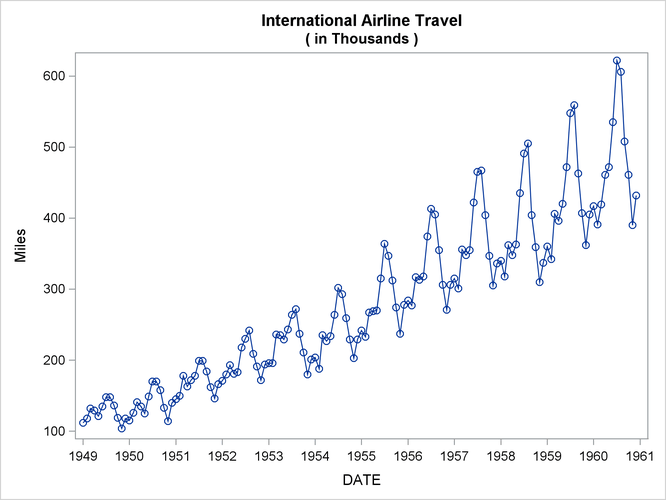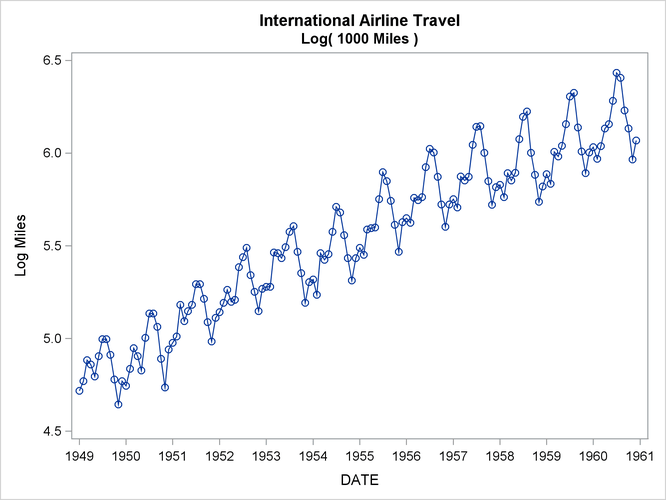Working with Time Series Data

Log Transformation

The logarithmic transformation is often useful for series that must be greater than zero and that grow exponentially. For example, Figure 3.15 shows a plot of an airline passenger miles series. Notice that the series has exponential growth and the variability of the series increases over time. Airline passenger miles must also be zero or greater.

Figure 3.15: Airline SeriesThe following statements compute the logarithms of the airline series:

```data lair;
set sashelp.air;
logair = log( air );
run;
```

Figure 3.16 shows a plot of the log-transformed airline series. Notice that the log series has a linear trend and constant variance.

Figure 3.16: Log Airline SeriesThe %LOGTEST macro can help you decide if a log transformation is appropriate for a series. See Chapter 5: SAS Macros and Functions, for more information about the %LOGTEST macro.